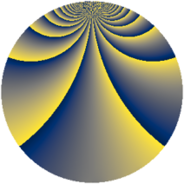# Properties

 Label 380.2.bbLevel $380$ Weight $2$ Character orbit 380.bb Rep. character $\chi_{380}(59,\cdot)$ Character field $\Q(\zeta_{18})$ Dimension $336$ Newform subspaces $1$ Sturm bound $120$ Trace bound $0$

# Related objects

## Defining parameters

 Level: $$N$$ $$=$$ $$380 = 2^{2} \cdot 5 \cdot 19$$ Weight: $$k$$ $$=$$ $$2$$ Character orbit: $$[\chi]$$ $$=$$ 380.bb (of order $$18$$ and degree $$6$$) Character conductor: $$\operatorname{cond}(\chi)$$ $$=$$ $$380$$ Character field: $$\Q(\zeta_{18})$$ Newform subspaces: $$1$$ Sturm bound: $$120$$ Trace bound: $$0$$

## Dimensions

The following table gives the dimensions of various subspaces of $$M_{2}(380, [\chi])$$.

Total New Old
Modular forms 384 384 0
Cusp forms 336 336 0
Eisenstein series 48 48 0

## Trace form

 $$336 q - 18 q^{4} - 12 q^{5} - 18 q^{6} - 24 q^{9} + O(q^{10})$$ $$336 q - 18 q^{4} - 12 q^{5} - 18 q^{6} - 24 q^{9} - 15 q^{10} + 18 q^{14} - 6 q^{16} - 42 q^{20} + 12 q^{21} + 12 q^{24} - 12 q^{25} + 18 q^{26} - 24 q^{29} - 24 q^{30} + 12 q^{34} - 6 q^{36} - 48 q^{40} - 12 q^{41} - 36 q^{44} - 6 q^{45} - 18 q^{46} - 108 q^{49} - 36 q^{50} + 36 q^{54} - 30 q^{60} - 24 q^{61} + 18 q^{64} - 18 q^{65} - 48 q^{66} - 180 q^{69} - 21 q^{70} - 30 q^{74} - 48 q^{76} + 3 q^{80} - 60 q^{81} + 90 q^{84} - 36 q^{85} + 102 q^{86} - 48 q^{89} - 78 q^{90} + 24 q^{96} + O(q^{100})$$

## Decomposition of $$S_{2}^{\mathrm{new}}(380, [\chi])$$ into newform subspaces

Label Dim $A$ Field CM Traces $q$-expansion
$a_{2}$ $a_{3}$ $a_{5}$ $a_{7}$
380.2.bb.a $336$ $3.034$ None $$0$$ $$0$$ $$-12$$ $$0$$